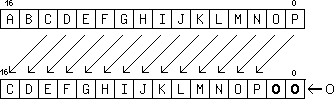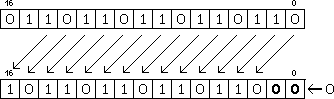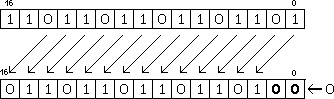version 2011-09-20

## SHL : logic SHift Left (multiply by a power of two)

The bits of the source register (snd) are shifted towards the MSB, by a number of bits indicated either by

The LSB of the result are filled with 0s.

In the following examples, we will shift a register by two positions. This amounts to a multiplication by 2^2=4. For YASEP16, the movement of the bits can be summarised by this diagram:For YASEP32, the principle is identical but with 32 bits instead of 16.

Let's consider that R1 gets loaded with 0110110110110110b, or 28086 in decimal. The left shifting operation can be written this way:```mov 0110110110110110b r1
shl 2 r1```
28086*4=112444, which does not fit in the register (limited to 65535) so the result is truncated and we get 112444%65536=46808=1011011011011000b

The sign of the result can change, if we consider signed integers, such as -9363, written 1101101101101101b in binary:This gives 0110110110110100b wich is the positive number 28084.